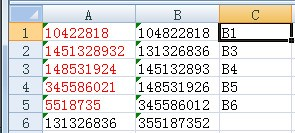• ZOL首页
• 产品报价
• 论坛
• ZOL问答
• 更多
• 手机客户端

• 回答数

4

• 浏览数

9,260

### 4个回答 默认排序 默认排序 按时间排序

=COUNTIF(\$B:\$B,A1)=0

=IF(COUNTIF(B:B,A1)=0,"B"&MAX((1-ISERROR(SEARCH(LEFT(A1,4),B:B))*ISERROR(SEARCH(RIGHT(A1,4),B:B)))*ROW(B:B)),"")C列中列出了对应A列的错误数据可能位于B列哪个单元格。

C1=IF(ISERROR(VLOOKUP(B1,A:A,1,0)),"",VLOOKUP(B1,A:A,1,0))

### 最新回答 更多>

ZOL问答 > 悬赏10分,求解:excel查找两列数据中 类似但是不完全相同(可能是输入错误)的数据,

### 举报成功